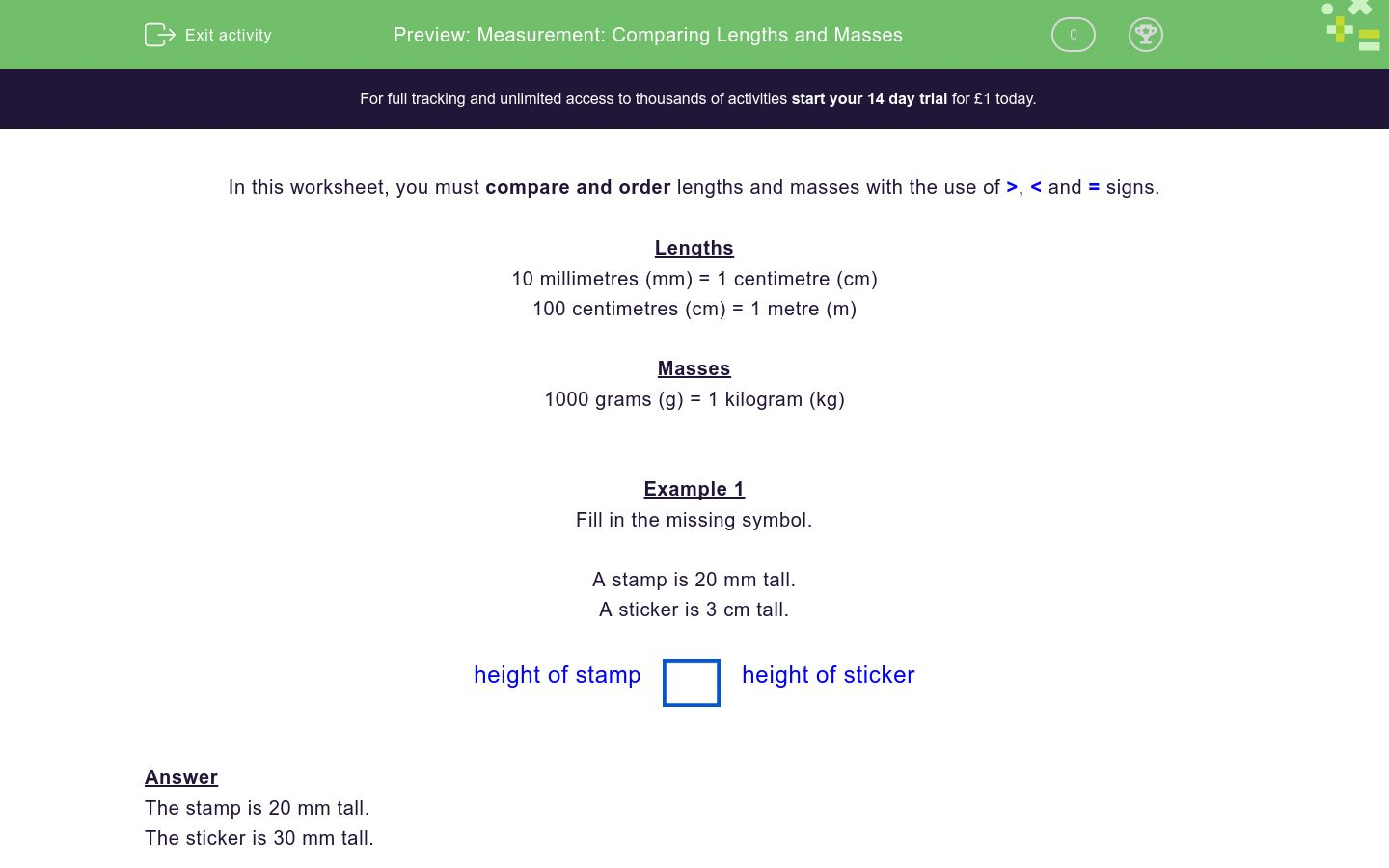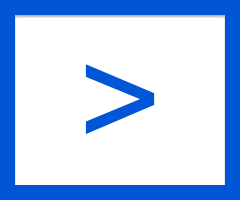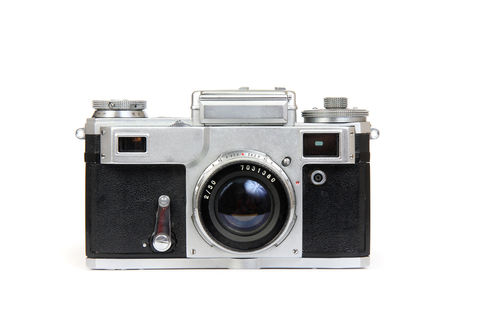# Measurement: Comparing Lengths and Masses

In this worksheet, students must compare and order lengths and masses, and must understand the use of >, < and = signs.Key stage:  KS 1

Curriculum topic:   Measurement

Curriculum subtopic:   Compare Measurements

Difficulty level:### QUESTION 1 of 10

In this worksheet, you must compare and order lengths and masses with the use of >< and = signs.

Lengths

10 millimetres (mm) = 1 centimetre (cm)

100 centimetres (cm) = 1 metre (m)

Masses

1000 grams (g) = 1 kilogram (kg)

Example 1

Fill in the missing symbol.

A stamp is 20 mm tall.

A sticker is 3 cm tall.

height of stampheight of sticker

The stamp is 20 mm tall.

The sticker is 30 mm tall.

20 is less than 30.

20 mm < 30 mm.

height of stampheight of sticker

Example 2

Fill in the missing symbol.

A packet of crisps has a mass of 200 g.

A bag of flour has a mass of 1 kg.

mass of flourmass of crisps

The crisps have a mass of 200 g.

The flour has a mass of 1000 g.

1000 is more than 200.

1000 g > 200 g.

mass of flourmass of crisps

Fill in the missing symbol.A stamp is 25 mm wide.

A sticker is 3 cm wide.

width of stampwidth of sticker

<

>

=

Fill in the missing symbol.A book has a mass of 550 g.

A calculator has a mass of 350 g.

mass of bookmass of calculator

<

>

=

Fill in the missing symbol.Fido       Rover      Happy

Fido is 35 cm tall.

Rover is 45 cm tall.

Happy is 55 cm tall.

Fido's heightHappy's height

<

>

=

Fill in the missing symbol.Fido       Rover      Happy

Fido has a mass of 22 kg.

Rover has a mass of 28 kg.

Happy has a mass of 30 kg.

Rover's massFido's mass

<

>

=

Fill in the missing symbol.A cake has a mass of 250 g.

A melon has a mass of 2 kg.

mass of melonmass of cake

<

>

=

Fill in the missing symbol.A giant book has a height of 50 cm.

A melon is ½ m tall.

height of melonheight of book

<

>

=

Fill in the missing symbol.A bicycle is 1 m tall.

4 year-old Jack is 100 cm tall.

Jack's heightheight of bicycle

<

>

=

Fill in the missing symbol.A camera has a mass of 550 g.

A cake has a mass of 1 kg.

mass of cameramass of cake

<

>

=

Fill in the missing symbol.A goldfish bowl is 30 cm tall.

1 brick is 10 cm tall.

height of bowlheight of 3 bricks

<

>

=

Fill in the missing symbol.A goldfish bowl has a mass of 1500 g.

3 bricks have a mass of 1 kg.

mass of bowlmass of 3 bricks

<

>

=

• Question 1

Fill in the missing symbol.A stamp is 25 mm wide.

A sticker is 3 cm wide.

width of stampwidth of sticker

<
EDDIE SAYS
25 mm < 30 mm
• Question 2

Fill in the missing symbol.A book has a mass of 550 g.

A calculator has a mass of 350 g.

mass of bookmass of calculator

>
EDDIE SAYS
550 g > 350 g
• Question 3

Fill in the missing symbol.Fido       Rover      Happy

Fido is 35 cm tall.

Rover is 45 cm tall.

Happy is 55 cm tall.

Fido's heightHappy's height

<
EDDIE SAYS
35 cm < 55 cm
• Question 4

Fill in the missing symbol.Fido       Rover      Happy

Fido has a mass of 22 kg.

Rover has a mass of 28 kg.

Happy has a mass of 30 kg.

Rover's massFido's mass

>
EDDIE SAYS
28 kg > 22 kg
• Question 5

Fill in the missing symbol.A cake has a mass of 250 g.

A melon has a mass of 2 kg.

mass of melonmass of cake

>
EDDIE SAYS
2000 g > 250 g
• Question 6

Fill in the missing symbol.A giant book has a height of 50 cm.

A melon is ½ m tall.

height of melonheight of book

=
EDDIE SAYS
½ metre = 50 cm
• Question 7

Fill in the missing symbol.A bicycle is 1 m tall.

4 year-old Jack is 100 cm tall.

Jack's heightheight of bicycle

=
EDDIE SAYS
1 metre = 100 cm
• Question 8

Fill in the missing symbol.A camera has a mass of 550 g.

A cake has a mass of 1 kg.

mass of cameramass of cake

<
EDDIE SAYS
550 g < 1000 g
• Question 9

Fill in the missing symbol.A goldfish bowl is 30 cm tall.

1 brick is 10 cm tall.

height of bowlheight of 3 bricks

=
EDDIE SAYS
Both are 30 cm tall.
• Question 10

Fill in the missing symbol.A goldfish bowl has a mass of 1500 g.

3 bricks have a mass of 1 kg.

mass of bowlmass of 3 bricks

>
EDDIE SAYS
1500 g > 1000 g
---- OR ----

Sign up for a £1 trial so you can track and measure your child's progress on this activity.

### What is EdPlace?

We're your National Curriculum aligned online education content provider helping each child succeed in English, maths and science from year 1 to GCSE. With an EdPlace account you’ll be able to track and measure progress, helping each child achieve their best. We build confidence and attainment by personalising each child’s learning at a level that suits them.

Get started